### BASELINE Statement

`BASELINE` <OUT=SAS-data-set> <OUTDIFF=SAS-data-set> <COVARIATES=SAS-data-set> <TIMELIST=list> <keyword=name …keyword=name> </ options> ;

The BASELINE statement creates a SAS data set (named by the OUT= option) that contains the baseline function estimates at the event times of each stratum for every set of covariates in the COVARIATES= data set. If the COVARIATES= data set is not specified, a reference set of covariates consisting of the reference levels for the CLASS variables and the average values for the continuous variables is used. You can use the DIRADJ option to obtain the direct adjusted survival curve that averages the estimated survival curves for the observations in the COVARIATES= data set. No BASELINE data set is created if the model contains a time-dependent variable defined by means of programming statement.

Table 67.2 summarizes the options available in the BASELINE statement.

Table 67.2: BASELINE Statement Options

Option

Description

Data Set and Time List Options

Specifies the output BASELINE data set

Specifies the output data set that contains differences of direct adjusted survival curves

Specifies the SAS data set that contains the explanatory variables

Specifies a list of time points for Bayesian computation of survival estimates.

Keyword Options

Specifies the cumulative mean function estimate

Specifies the cumulative hazard function estimate

Specifies the log of the negative log of SURVIVAL

Specifies the log of SURVIVAL

Specifies the lower pointwise confidence limit for CMF

specifies the lower pointwise confidence limit for CUMHAZ

LOWERHPDCUMHAZ=

Specifies the lower limit of the HPD interval for CUMHAZ

Specifies the lower limit of the HPD interval for SURVIVAL

Specifies the lower pointwise confidence limit for SURVIVAL

Specifies the estimated standard error of CMF

Specifies the estimated standard error of CUMHAZ

Specifies the standard error of SURVIVAL

Specifies the estimated standard error of the linear predictor estimator

Specifies the survivor function estimate

Specifies the upper pointwise confidence limit for CMF

Specifies the upper pointwise confidence limit for CUMHAZ

UPPERHPDCUMHAZ=

Specifies the upper limit of the HPD interval for CUMHAZ

Specifies the upper limit of the HPD interval for SURVIVAL

Specifies the upper pointwise confidence limit for SURVIVAL

Specifies the estimate of the linear predictor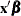Other Options

Specifies the significance level of the confidence interval for the survivor function

Specifies the method used to compute the confidence limits

Names a variable whose values are used to identify or group the survival curves

Specifies the method used to compute the survivor function estimates

Names the variable in the COVARIATES= data set for identifying the baseline functions curves in the plots

The following options are available in the BASELINE statement.

OUT=SAS-data-set

names the output BASELINE data set. If you omit the OUT= option, the data set is created and given a default name by using the `DATAn` convention. See the section OUT= Output Data Set in the BASELINE Statement for more information.

OUTDIFF=SAS-data-set

names the output data set that contains all pairwise differences of direct adjusted probabilities between groups if the GROUP= variable is specified, or between strata if the GROUP= variable is not specified. It is required that the DIRADJ option be specified to use the OUTDIFF= option.

COVARIATES=SAS-data-set

names the SAS data set that contains the sets of explanatory variable values for which the quantities of interest are estimated. All variables in the COVARIATES= data set are copied to the OUT= data set. Thus, any variable in the COVARIATES= data set can be used to identify the covariate sets in the OUT= data set.

TIMELIST=list

specifies a list of time points at which the survival function estimates and cumulative hazard function estimates are computed. The following specifications are equivalent:

```   timelist=5,20 to 50 by 10
timelist=5 20 30 40 50
```

If the TIMELIST= option is not specified, the default is to carry out the prediction at all event times and at time 0. This option can be used only for the Bayesian analysis.

keyword=name

specifies the statistics to be included in the OUT= data set and assigns names to the variables that contain these statistics. Specify a keyword for each desired statistic, an equal sign, and the name of the variable for the statistic. Not all keywords listed in Table 67.3 (and discussed in the text that follows) are appropriate for both the classical analysis and the Bayesian analysis; and the table summaries the choices for each analysis.

Table 67.3: Summary of the Keyword Choices

Keyword

Classical

Bayesian

Survivor Function

x

x

x

x

x

x

x

x

x

x

Cumulative Hazard Function

x

x

x

x

x

x

x

x

x

x

Cumulative Mean Function

x

x

x

x

Others

x

x

x

x

x

x

The available keywords are as follows.

CMF=
MCF=

specifies the cumulative mean function estimate for recurrent events data. Specifying CMF=_ALL_ is equivalent to specifying CMF=CMF, STDCMF=StdErrCMF, LOWERCMF=LowerCMF, and UPPERCMF=UpperCMF. Nelson (2002) refers to the mean function estimate as MCF (mean cumulative function).

CUMHAZ=

specifies the cumulative hazard function estimate. Specifying CUMHAZ=_ALL_ is equivalent to specifying CUMHAZ=CumHaz, STDCUMHAZ=StdErrCumHaz, LOWERCUMHAZ=LowerCumHaz, and UPPERCUMHAZ=UpperCumHaz. For a Bayesian analysis, CUMHAZ=_ALL_ also includes LOWERHPDCUMHAZ= LowerHPDCumHaz and UpperHPDCUMHAZ=UpperHPDCumHaz.

LOGLOGS=

specifies the log of the negative log of SURVIVAL.

LOGSURV=

specifies the log of SURVIVAL.

LOWER=
L=

specifies the lower pointwise confidence limit for the survivor function. For a Bayesian analysis, this is the lower limit of the equal-tail credible interval for the survivor function. The confidence level is determined by the ALPHA= option.

LOWERCMF=
LOWERMCF=

specifies the lower pointwise confidence limit for the cumulative mean function. The confidence level is determined by the ALPHA= option.

LOWERHPD=

specifies the lower limit of the HPD interval for the survivor function. The confidence level is determined by the ALPHA= option.

LOWERHPDCUMHAZ=

specifies the lower limit of the HPD interval for the cumulative hazard function. The confidence level is determined by the ALPHA= option.

LOWERCUMHAZ=

specifies the lower pointwise confidence limit for the cumulative hazard function. For a Bayesian analysis, this is the lower limit of the equal-tail credible interval for the cumulative hazard function. The confidence level is determined by the ALPHA= option.

STDERR=

specifies the standard error of the survivor function estimator. For a Bayesian analysis, this is the standard deviation of the posterior distribution of the survivor function.

STDCMF=
STDMCF=

specifies the estimated standard error of the cumulative mean function estimator.

STDCUMHAZ=

specifies the estimated standard error of the cumulative hazard function estimator. For a Bayesian analysis, this is the standard deviation of the posterior distribution of the cumulative hazard function.

STDXBETA=

specifies the estimated standard error of the linear predictor estimator. For a Bayesian analysis, this is the standard deviation of the posterior distribution of the linear predictor.

SURVIVAL=

specifies the survivor function (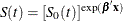) estimate. Specifying SURVIVAL=_ALL_ is equivalent to specifying SURVIVAL=Survival, STDERR=StdErrSurvival, LOWER=LowerSurvival, and UPPER=UpperSurvival; and for a Bayesian analysis, SURVIVAL=_ALL_ also specifies LOWERHPD= LowerHPDSurvival and UPPERHPD=UpperHPDSurvival.

UPPER=
U=

specifies the upper pointwise confidence limit for the survivor function. For a Bayesian analysis, this is the upper limit of the equal-tail credible interval for the survivor function. The confidence level is determined by the ALPHA= option.

UPPERCMF=
UPPERMCF=

specifies the upper pointwise confidence limit for the cumulative mean function. The confidence level is determined by the ALPHA= option.

UPPERCUMHAZ=

specifies the upper pointwise confidence limit for the cumulative hazard function. For a Bayesian analysis, this is the upper limit of the equal-tail credible interval for the cumulative hazard function. The confidence level is determined by the ALPHA= option.

UPPERHPD=

specifies the upper limit of the equal-tail credible interval for the survivor function. The confidence level is determined by the ALPHA= option.

UPPERHPDCUMHAZ=

specifies the upper limit of the equal-tail credible interval for the cumulative hazard function. The confidence level is determined by the ALPHA= option.

XBETA=

specifies the estimate of the linear predictor.

The following options can appear in the BASELINE statement after a slash (/). The METHOD= and CLTYPE= options apply only to the estimate of the survivor function in the classical analysis. For the Bayesian analysis, the survivor function is estimated by the Breslow (1972) method.

ALPHA=value

specifies the significance level of the confidence interval for the survivor function. The value must be between 0 and 1. The default is the value of the ALPHA= option in the PROC PHREG statement, or 0.05 if that option is not specified.

CLTYPE=method

specifies the method used to compute the confidence limits for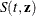, the survivor function for a subject with a fixed covariate vectorat event time t. The CLTYPE= option can take the following values:

LOG

specifies that the confidence limits for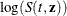be computed using the normal theory approximation. The confidence limits forare obtained by back-transforming the confidence limits for. The default is CLTYPE=LOG.

LOGLOG

specifies that the confidence limits for the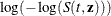be computed using normal theory approximation. The confidence limits forare obtained by back-transforming the confidence limits for.

NORMAL

<LINEAR> specifies that the confidence limits forbe computed directly using normal theory approximation.

computes direct adjusted survival curves (Makuch, 1982; Gail and Byar, 1986; Zhang et al., 2007) by averaging the estimated survival curves for the observations in the COVARIATES= data set. See the section Direct Adjusted Survival Curves and Example 67.8 for the computation and specific details. If the COVARIATES= data set is not specified, the input data set specified in the DATA= option in the PROC PHREG statement is used instead. If you also specify the GROUP= option, PROC PHREG computes an adjusted survival curve for each value of the GROUP= variable.

GROUP=variable

names a variable whose values identify or group the estimated survival curves. The behavior of this option depends on whether you also specify the DIRADJ option:

• If you also specify the DIRADJ option, variable must be a CLASS variable in the model. A direct adjusted survival curve is computed for each value of variable in the input data. The variable does not have to be a variable in the COVARIATES= data set. Each direct adjusted survival curve is the average of the survival curves of all individuals in the COVARIATES= data set with their value of variable set to a specific value.

• If you do not specify the DIRADJ option, variable is required to be a numeric variable in the COVARIATES= data set. Survival curves for the observations with the same value of the variable are overlaid in the same plot.

METHOD=method

specifies the method used to compute the survivor function estimates. See the section Survivor Function Estimators for details. You can specify the following methods:

BRESLOW
CH
EMP

specifies that the Breslow (1972) estimator be used to compute the survivor function—that is, that the survivor function be estimated by exponentiating the negative empirical cumulative hazard function.

FH

specifies that the Fleming-Harrington (FH) estimates be computed. The FH estimator is a tie-breaking modification of the Breslow estimator. If there are no tied event times, this estimator is the same as the Breslow estimator.

PL

specifies that the product-limit estimates of the survivor function be computed. This estimator is not available if you use the model syntax that allows two time variables for counting process style of input; in such a case the Breslow estimator (METHOD=BRESLOW) is used instead.

The default is METHOD=BRESLOW.

ROWID=variable
ID=variable
ROW=variable

names a variable in the COVARIATES= data set for identifying the baseline function curves in the plots. This option has no effect if the PLOTS= option in the PROC PHREG statement is not specified. Values of this variable are used to label the curves for the corresponding rows in the COVARIATES= data set. You can specify ROWID=_OBS_ to use the observation numbers in the COVARIATES= data set for identification.

For recurrent events data, both CMF= and CUMHAZ= statistics are the Nelson estimators, but their standard error are not the same. Confidence limits for the cumulative mean function and cumulative hazard function are based on the log transform.